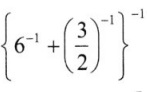# Grade 8 Exponents and Powers Worksheets

## Grade 8 Maths Exponents and Powers Multiple Choice Questions (MCQs)

1. Which one is greater?
(a) 23
(b) 32
(c) 18
(d) 42

2. The value of 2 is:
(a) 128
(b) 256
(c) 512
(d) None of these

3. The exponent in the expression 37 is ……………… .
(a) 1
(b) 7
(c) 0
(d) 3

4. The value of 3° is …………….. .
(a) 0
(b) 3
(c) 1
(d) None of these

5. Multiplicative inverse of$\frac{1}{7}$ is ……………….. .
(a) 49
(b) 5
(c) 7
(d) -14

6. Fill in the blank: am ÷ an = a. Where m and n are natural number:
(a) mn
(b) m + n
(c) m – n
(d) m ÷ n

7. The value of$\frac{1}{3^{2}}$ is equal to ……………. .
(a)$\frac{1}{9}$
(b) 1
(c) -6
(d)$\frac{1}{3}$

8. In simplified form (3° + 4° + 5°)° is equals to:
(a) 12
(b) 3
(c) 12
(d) 1

9. 1 micron is equal to ……………. .
(a)$\frac{1}{100000}$ m
(b) 106 m
(c) 105 m
(d) 107 m

10. The approximate distance of moon from the earth is 384467000 m and in exponential form this distance can be written as ………………. .
(a) 3.84467 × 108 m
(b) 384467 × 10-8m
(c) 384467 × 10-9m
(d) 3.84467 × 10-3 m

11. 7 × 10-5 m is the standard form of which of the following …………….. .
(a) 0.0007 m
(b) 0.000007 m
(c) 0.0000007 m
(d) 0.00007 m

12. The expression, (52 + 72 + 32)0 is equals to:
(a) 156
(b) -6
(c) 1
(d) 83

13. The value of$\left(\frac{1}{6}\right)^{2}$ is ……………… .
(a)$\frac{1}{12}$
(b)$\frac{2}{3}$
(c)$\frac{1}{3}$
(d) 2

14. The value of (6-1 – 8-1)-1 is:
(a)$\frac{-1}{12}$
(b) -2
(c)$\frac{1}{24}$
(d) 24

15. The value ofis:
(a)$\frac{2}{3}$
(b)$\frac{5}{6}$
(c)$\frac{6}{5}$
(d) None of these

16. The value of$\left[\frac{-1}{2}\right]^{-6}$ is:
(a) -64
(b)$\frac{-1}{64}$
(c)$\frac{1}{64}$
(d) 64

17. The value of (32 – 22) ×$\left(\frac{2}{3}\right)^{-3}$ is:
(a)$\frac{45}{8}$
(b)$\frac{135}{8}$
(c)$\frac{8}{135}$
(d)$\frac{8}{45}$

18. If, then the value of x is:
(a) -1
(b)$\frac{1}{2}$
(c)$\frac{-1}{2}$
(d) None of these

19. If 52x+1 ÷ 25 = 125, then the value of x is:
(a) 2
(b)$\frac{1}{2}$
(c)$\frac{-1}{2}$
(d) -2

20. If (23x-1 + 10) ÷ 7 = 6, then the value of x is:
(a) 2
(b) 0
(c) 1
(d) -2

### Class 8 Maths Exponents and Powers Fill In The Blanks

1. The value of 34 = ………………..
2. 125° + 52 = ………………
3. Multiplicative inverse of 2-5 = ………………..
4. 114 × 11-2 = ………………….
5.$\frac{1}{7^{-4}}$ = …………………
6. The value of (-1)55 is ………………….
7. The value of (-1)500 is ……………….
8. (-1)even number = ………………
9. In standard form 56700000 is written as ………………..
10. Usual form of the expression 9 × 105 is given by ……………..

### Class 8 Maths Exponents and Powers Very Short Answer Type Questions

1. Express 729 as a power of 3.
2. Express 2048 as a power 2.
3. Simplify and write in exponential form of 22 × 25.
4. Simplify and write in exponential form of (-4)100 × (-4)20
5. Simplify and write in exponential form of 52 × 57 × 512
6. Find the number from the following expanded form : 9 × 105 + 2 × 102 + 3 × 101

### Class 8 Maths Exponents and Powers Short Answer Type Questions

1. Simplify:2. Simplify:3. If 3x = 243, then find the value of x.
4. Simplify:### Class 8 Maths Exponents and Powers Long Answer Type Questions

1. What is the multiplicative inverse of (64)2/3 ×$\left(\frac{1}{4}\right)^{-3}$
2. Find the value of x if (-3)3x+1 × (-3)4 = (-3)8
3. Express the height of bundle of 500 papers placed on each other if thickness of one paper is 0.0016 cm.